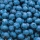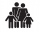# Latest word problems

#### Number of problems found: 633

• The trapeziumThe trapezium is formed by cutting the top of the right-angled isosceles triangle. The base of the trapezium is 10 cm and the top is 5 cm. Find the area of trapezium.
• Blueberries5 children collect 4 liters of blueberries in 1.5 hours. a) How many minutes do 3 children take 2 liters of blueberries? b) How many liters of blueberries will be taken by 8 children in 3 hours?
• ChocolateChocolate, which originally cost 1.5 euros, was increased by 40%. How many euros did it cost?
• Father and sonsFather is 27 and his sons 2 and one year. In how many years will his sons sum up to half his age?
• Three linesAt 6 am, three bus lines are departing from the station. The first line has an interval of 24 minutes. The second line has an interval of 15 minutes. The third line runs at regular intervals of more than 1 minute. The third line runs at the same time as tFind the radius of the circle with area S = 200 cm².
• Evaluate - order of opsEvaluate the expression: 32+2[5×(24-6)]-48÷24 Pay attention to the order of operation including integers
• Sides of right angled triangleOne leg is 1 m shorter than the hypotenuse, and the second leg is 2 m shorter than the hypotenuse. Find the lengths of all sides of the right-angled triangle.
• Before yesterdayHe merchant adds a sale sign in his shop window to the showed pair of shoes in the morning: "Today by p% cheaper than yesterday. " After a while, however, he decided that the sign saying: "Today 62.5% cheaper than the day before yesterday". Determine the
• Coordinates of square verticesI have coordinates of square vertices A / -3; 1/and B/1; 4 /. Find coordinates of vertices C and D, C 'and D'. Thanks Peter.
• A rhombusA rhombus has sides of length 10 cm, and the angle between two adjacent sides is 76 degrees. Find the length of the longer diagonal of the rhombus.
• Positional energyWhat velocity in km/h must a body weighing 60 kg have for its kinetic energy to be the same as its positional energy at the height 50 m?
• Isosceles triangle 10In an isosceles triangle, the equal sides are 2/3 of the length of the base. Determine the measure of the base angles.

We apologize, but in this category are not a lot of examples.
Do you have an interesting mathematical word problem that you can't solve it? Submit a math problem, and we can try to solve it.

We will send a solution to your e-mail address. Solved examples are also published here. Please enter the e-mail correctly and check whether you don't have a full mailbox.

Please do not submit problems from current active competitions such as Mathematical Olympiad, correspondence seminars etc...